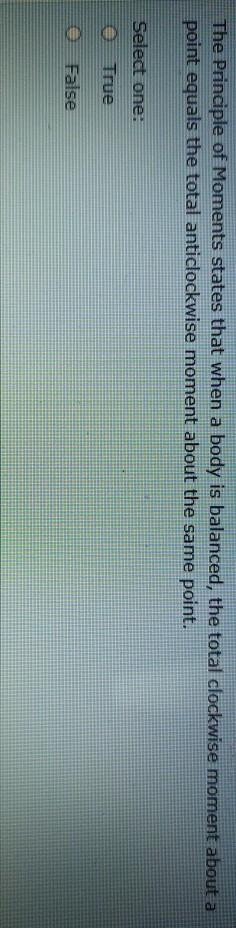# Question The Principle of Moments states that when a body is balanced, the total clockwise moment about a point equals the total anticlockwise moment about the same point. Select one: True FalseWZMLBS The Asker · Civil EngineeringTranscribed Image Text: The Principle of Moments states that when a body is balanced, the total clockwise moment about a point equals the total anticlockwise moment about the same point. Select one: True False
More
Transcribed Image Text: The Principle of Moments states that when a body is balanced, the total clockwise moment about a point equals the total anticlockwise moment about the same point. Select one: True False